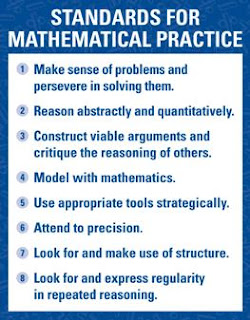## Thursday, August 22, 2013

### Common Core Standards for Mathematical Practice

Guest Blog Post by Kitty Rutherford

The Standards for Mathematical Practice impact all math teaching and learning. They focus on what it means for students to be mathematically proficient.  I have heard many say the Standards for Mathematical Practice are the heart and soul of the Common Core State Standards. These standards describe student behaviors, ensure an understanding of math, focus on the development of reasoning, and building mathematical communication.  Each standard has a unique focus, but each standard is also interwoven with the others as we put them into practice. These practices empower students to use math and to think mathematically. Our job as a teacher is to help students develop these practices to become effective mathematicians.

The Standards for Mathematical Practice describe varieties of expertise that mathematics educators at all levels should seek to develop in their students. These practices rest on important “processes and proficiencies” with longstanding importance in mathematics education. The first of these are the NCTM process standards of problem solving, reasoning and proof, communication, representation, and connections. The second are the strands of mathematical proficiency specified in the National Research Council’s report Adding It Up: adaptive reasoning, strategic competence, conceptual understanding (comprehension of mathematical concepts, operations and relations), procedural fluency (skill in carrying out procedures flexibly, accurately, efficiently and appropriately), and productive disposition (habitual inclination to see mathematics as sensible, useful, and worthwhile, coupled with a belief in diligence and one’s own efficacy).The Standards for Mathematical Practice are a significant focus of Common Core State Standards. These eight practices describe the thinking processes, habits of mind, and dispositions that students need to develop a deep, flexible, and enduring understanding of mathematics.

One way to build a deep understanding of the Standards for Mathematical Practice is to read one practice at a time and reflect on the following questions:
1. Why is this practice important?
2. What does this practice look like when students are doing it?
3. How can a teacher model this practice?
4. What could a teacher do within a lesson to encourage students in this practice?
5. How can you assess proficiency in this practice?

Before Common Core State Standards were implemented, I visited a few fourth grade mathematics classrooms.  I provided a high-leverage math task, students worked collaboratively together to solve.  I then introduced the Standards for Mathematical Practice by explaining to the students that these standards were practices that mathematicians do to help them excel in mathematical thinking. I asked them to think about the task they just solved, if they were to explain to someone what that practice meant or looked like how would they do it.

After some discussion, students created posters to represent their thinking about the Mathematical Practices. In this task students explored prime or composite by building possible rectangular arrays for numbers 1-25.

### These are some of the posters students created after the task and much discussion.

Which products are composite and which are prime? I’ll think of products that have one factor pair and which have more than one factor pair.

This number is prime so it only has 1 array for the number.
This number has 2 arrays so it must be composite.
Using correct math language to explain your thinking is important

In another fourth grade classroom students were given the task of finding the perimeter of a figure. These were the posters of the Standards for Mathematical Practice they created.

Yes
Cha-Ching

#### Model with Mathematics.

If I’m going to tile the perimeter, how many pieces of tile will I need?
Do these side lengths make sense?

#### Model with Mathematics.

These color tiles help me to find the missing sides so I can write an equation to find the perimeter.

I noticed a blond-hair, blue eyed, little boy working diligently by himself, as I advanced towards him, I noticed his simplistic drawing (see below).  As I approached, he looked up and in a sincere heart-felt tone he informed me that this was a picture of his friend persevering not only in math but in his everyday life. Pointing to the empty desk next to his, he whispered, “My friend has cancer. He is not in school because he just received a cancer treatment a few days ago.” His comments shook me to the very core. “Many of these Practices we do every day, not just in Mathematics!"

What do I need to know to solve?
This will be easy!

Kitty Rutherford serves as the North Carolina Elementary Mathematics Consultant for the Department of Public Instruction in Raleigh. She is an experienced leader, collaborator and licensed educator with a Master’s Degree in Elementary Education. She has received many honors such as the Presidential Award for Excellence in Mathematics and Science Teaching and the NCCTM Outstanding Elementary Mathematics Teacher. She is a member of the NCAEE Board and will be presenting two sessions at that Elementary School Conference. For more resources for implementing Common Core Standards, visit the Mathematics Wiki hosted by the NC DPI.

1.1.2.